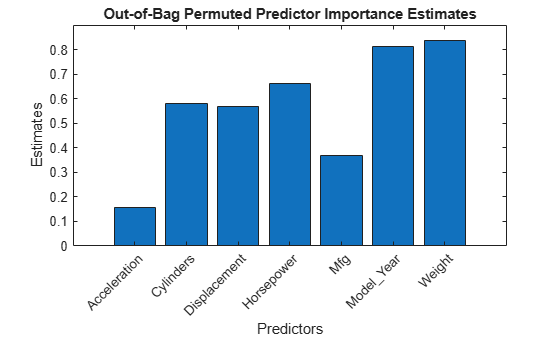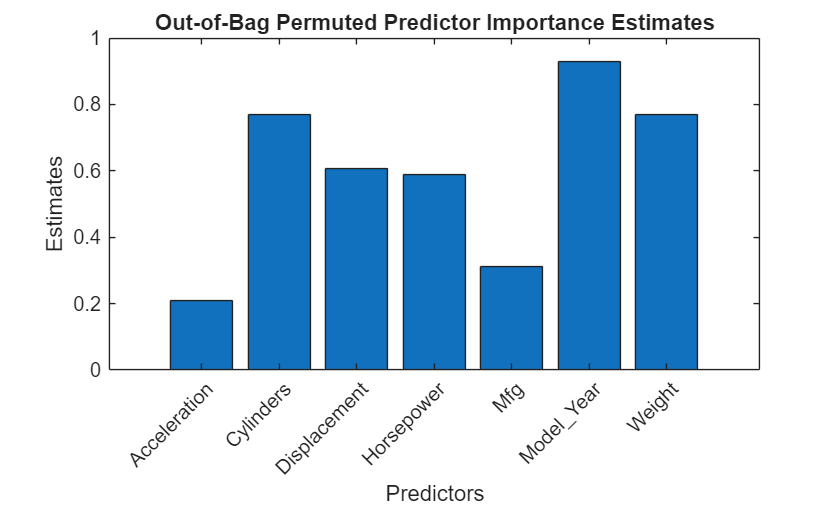# oobPermutedPredictorImportance

Predictor importance estimates by permutation of out-of-bag predictor observations for random forest of regression trees

## Syntax

``Imp = oobPermutedPredictorImportance(Mdl)``
``Imp = oobPermutedPredictorImportance(Mdl,Name,Value)``

## Description

example

````Imp = oobPermutedPredictorImportance(Mdl)` returns a vector of out-of-bag, predictor importance estimates by permutation using the random forest of regression trees `Mdl`. `Mdl` must be a `RegressionBaggedEnsemble` model object.```

example

````Imp = oobPermutedPredictorImportance(Mdl,Name,Value)` uses additional options specified by one or more `Name,Value` pair arguments. For example, you can speed up computation using parallel computing or indicate which trees to use in the predictor importance estimation.```

## Input Arguments

expand all

Random forest of regression trees, specified as a `RegressionBaggedEnsemble` model object created by `fitrensemble`.

### Name-Value Pair Arguments

Specify optional comma-separated pairs of `Name,Value` arguments. `Name` is the argument name and `Value` is the corresponding value. `Name` must appear inside quotes. You can specify several name and value pair arguments in any order as `Name1,Value1,...,NameN,ValueN`.

Indices of learners to use in predictor importance estimation, specified as the comma-separated pair consisting of `'Learners'` and a numeric vector of positive integers. Values must be at most `Mdl.NumTrained`. When `oobPermutedPredictorImportance` estimates the predictor importance, it includes the learners in `Mdl.Trained(learners)` only, where `learners` is the value of `'Learners'`.

Example: `'Learners',[1:2:Mdl.NumTrained]`

Parallel computing options, specified as the comma-separated pair consisting of `'Options'` and a structure array returned by `statset`. `'Options'` requires a Parallel Computing Toolbox™ license.

`oobPermutedPredictorImportance` uses the `'UseParallel'` field only. `statset('UseParallel',true)` invokes a pool of workers.

Example: `'Options',statset('UseParallel',true)`

## Output Arguments

expand all

Out-of-bag, predictor importance estimates by permutation, returned as a 1-by-p numeric vector. p is the number of predictor variables in the training data (`size(Mdl.X,2)`). `Imp(j)` is the predictor importance of the predictor `Mdl.PredictorNames(j)`.

## Examples

expand all

Load the `carsmall` data set. Consider a model that predicts the mean fuel economy of a car given its acceleration, number of cylinders, engine displacement, horsepower, manufacturer, model year, and weight. Consider `Cylinders`, `Mfg`, and `Model_Year` as categorical variables.

```load carsmall Cylinders = categorical(Cylinders); Mfg = categorical(cellstr(Mfg)); Model_Year = categorical(Model_Year); X = table(Acceleration,Cylinders,Displacement,Horsepower,Mfg,... Model_Year,Weight,MPG);```

You can train a random forest of 500 regression trees using the entire data set.

```Mdl = fitrensemble(X,'MPG','Method','Bag','NumLearningCycles',500); ```

`fitrensemble` uses a default template tree object `templateTree()` as a weak learner when `'Method'` is `'Bag'`. In this example, for reproducibility, specify `'Reproducible',true` when you create a tree template object, and then use the object as a weak learner.

```rng('default') % For reproducibility t = templateTree('Reproducible',true); % For reproducibiliy of random predictor selections Mdl = fitrensemble(X,'MPG','Method','Bag','NumLearningCycles',500,'Learners',t);```

`Mdl` is a `RegressionBaggedEnsemble` model.

Estimate predictor importance measures by permuting out-of-bag observations. Compare the estimates using a bar graph.

```imp = oobPermutedPredictorImportance(Mdl); figure; bar(imp); title('Out-of-Bag Permuted Predictor Importance Estimates'); ylabel('Estimates'); xlabel('Predictors'); h = gca; h.XTickLabel = Mdl.PredictorNames; h.XTickLabelRotation = 45; h.TickLabelInterpreter = 'none';````imp` is a 1-by-7 vector of predictor importance estimates. Larger values indicate predictors that have a greater influence on predictions. In this case, `Weight` is the most important predictor, followed by `Model_Year`.

Load the `carsmall` data set. Consider a model that predicts the mean fuel economy of a car given its acceleration, number of cylinders, engine displacement, horsepower, manufacturer, model year, and weight. Consider `Cylinders`, `Mfg`, and `Model_Year` as categorical variables.

```load carsmall Cylinders = categorical(Cylinders); Mfg = categorical(cellstr(Mfg)); Model_Year = categorical(Model_Year); X = table(Acceleration,Cylinders,Displacement,Horsepower,Mfg,... Model_Year,Weight,MPG);```

Display the number of categories represented in the categorical variables.

`numCylinders = numel(categories(Cylinders))`
```numCylinders = 3 ```
`numMfg = numel(categories(Mfg))`
```numMfg = 28 ```
`numModelYear = numel(categories(Model_Year))`
```numModelYear = 3 ```

Because there are 3 categories only in `Cylinders` and `Model_Year`, the standard CART, predictor-splitting algorithm prefers splitting a continuous predictor over these two variables.

Train a random forest of 500 regression trees using the entire data set. To grow unbiased trees, specify usage of the curvature test for splitting predictors. Because there are missing values in the data, specify usage of surrogate splits. To reproduce random predictor selections, set the seed of the random number generator by using `rng` and specify `'Reproducible',true`.

```rng('default'); % For reproducibility t = templateTree('PredictorSelection','curvature','Surrogate','on', ... 'Reproducible',true); % For reproducibility of random predictor selections Mdl = fitrensemble(X,'MPG','Method','bag','NumLearningCycles',500, ... 'Learners',t);```

Estimate predictor importance measures by permuting out-of-bag observations. Perform calculations in parallel.

```options = statset('UseParallel',true); imp = oobPermutedPredictorImportance(Mdl,'Options',options);```
```Starting parallel pool (parpool) using the 'local' profile ... Connected to the parallel pool (number of workers: 6). ```

Compare the estimates using a bar graph.

```figure; bar(imp); title('Out-of-Bag Permuted Predictor Importance Estimates'); ylabel('Estimates'); xlabel('Predictors'); h = gca; h.XTickLabel = Mdl.PredictorNames; h.XTickLabelRotation = 45; h.TickLabelInterpreter = 'none';```In this case, `Model_Year` is the most important predictor, followed by `Cylinders`. Compare these results to the results in Estimate Importance of Predictors.

expand all

## Tips

When growing a random forest using `fitrensemble`:

• Standard CART tends to select split predictors containing many distinct values, e.g., continuous variables, over those containing few distinct values, e.g., categorical variables . If the predictor data set is heterogeneous, or if there are predictors that have relatively fewer distinct values than other variables, then consider specifying the curvature or interaction test.

• Trees grown using standard CART are not sensitive to predictor variable interactions. Also, such trees are less likely to identify important variables in the presence of many irrelevant predictors than the application of the interaction test. Therefore, to account for predictor interactions and identify importance variables in the presence of many irrelevant variables, specify the interaction test .

• If the training data includes many predictors and you want to analyze predictor importance, then specify `'NumVariablesToSample'` of the `templateTree` function as `'all'` for the tree learners of the ensemble. Otherwise, the software might not select some predictors, underestimating their importance.

For more details, see `templateTree` and Choose Split Predictor Selection Technique.

## References

 Breiman, L., J. Friedman, R. Olshen, and C. Stone. Classification and Regression Trees. Boca Raton, FL: CRC Press, 1984.

 Loh, W.Y. “Regression Trees with Unbiased Variable Selection and Interaction Detection.” Statistica Sinica, Vol. 12, 2002, pp. 361–386.

 Loh, W.Y. and Y.S. Shih. “Split Selection Methods for Classification Trees.” Statistica Sinica, Vol. 7, 1997, pp. 815–840.